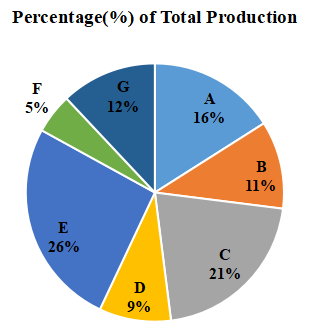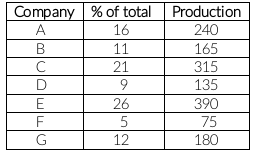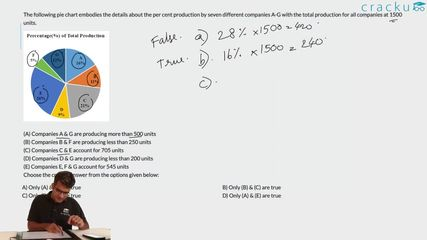Question 8

# The following pie chart embodies the details about the per cent production by seven different companies A-G with the total production for all companies at 1500 units.(A) Companies A & G are producing more than 500 units(B) Companies B & F are producing less than 250 units(C) Companies C & E account for 705 units(D) Companies D & G are producing less than 200 units(E) Companies E, F & G account for 545 unitsChoose the correct answer from the options given below:

Solution

Total production = 1500 unitsA) A and G production = 240 + 180 = 420 (not greater than 500)

B) B and F production = 165 + 75 = 240 ( is less than 250)

C) C and E production = 315 + 390 = 705

D) D and G production = 135 +180 = 315 ( not less than 200)

E) E, F and G production = 390 + 75 + 180 = 645 ( not equal to 545)

Only (B) and (C) are true.

### View Video Solution